# Analog Filter Design Demystified

### Abstract

This article shows how to design analog filters. It starts by covering the fundamentals of filters, goes on to introduce the basic types like Butterworth, Chebyshev, and Bessel, and then guides the reader through the design process for lowpass and highpass filters. Included are the derivation of the equations and the circuit implementation.

It's a jungle out there.

A small tribe, in the dense wilderness, is much sought after by head hunters from the surrounding plains. Known throughout the land for their esoteric expertise, this is the tribe of the Analog Engineers, who live in the farthest regions of the left half Plains, past the jungles of Laplace.

The guru of analog engineers is the Analog Filter Designer, who sits on the throne of his kingdom and imparts wisdom. You never get to see him, even with an appointment, and you call him "Sir."

The countless pages of equations found in most books on filter design can frighten small dogs, and digital designers. This article clears a path through the brush for the practical engineer and unravels the mystery of filter design, enabling you to design continuous-time analog filters quickly and with a minimum of mathematics.

### The Theory of Analog Electronics

Analog electronics has two distinct sides: the theory taught by academic institutions (equations of stability, phase-shift calculations, etc.), and the practical side familiar to most engineers (avoid oscillation by tweaking the gain with a capacitor, etc.). Unfortunately, filter design is based firmly on long-established equations and tables of theoretical results. Filter design from theoretical equations can prove arduous. Consequently, this discussion employs a minimum of math—either in translating the theoretical tables into practical component values, or in deriving the response of a general-purpose filter.

### The Fundamentals

Simple RC lowpass filters have the transfer function: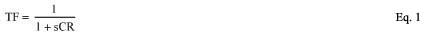Cascading such filters complicates the response by giving rise to quadratic equations in the denominator of the transfer function. Thus, the denominator of the transfer function for any second-order lowpass filter is as2+ bs + c. Substituting values for a, b and c determines the filter response over frequency. Anyone who remembers high school math will note that the above expression equals zero for certain values of "s" given by the equation:At the values of "s" for which this quadratic equation equals zero, the transfer function has theoretically infinite gain. These values, which establish the performance of each type of filter over frequency, are known as the poles of the quadratic equation. Poles usually occur as pairs, in the form of a complex number (a + jb) and its complex conjugate (a - jb). The term jb is sometimes zero.

The thought of a transfer function with infinite gain may frighten nervous readers, but in practice it isn't a problem. The pole's real part "a" indicates how the filter responds to transients, and its imaginary part "jb" indicates the response over frequency. As long as this real part is negative, the system is stable. The following text explains how to transfer the tables of poles found in many text books into component values suitable for circuit design.

### Filter Types

The most common filter responses are the Butterworth, Chebyshev, and Bessel types. Many other types are available, but 90% of all applications can be solved with one of these three. Butterworth ensures a flat response in the passband and an adequate rate of rolloff. A good "all rounder," the Butterworth filter is simple to understand and suitable for applications such as audio processing. The Chebyshev gives a much steeper rolloff, but passband ripple makes it unsuitable for audio systems. It is superior for applications in which the passband includes only one frequency of interest (e.g., the derivation of a sine wave from a square wave, by filtering out the harmonics).

The Bessel filter gives a constant propagation delay across the input frequency spectrum. Therefore, applying a square wave (consisting of a fundamental and many harmonics) to the input of a Bessel filter yields an output square wave with no overshoot (all the frequencies are delayed by the same amount). Other filters delay the harmonics by different amounts, resulting in an overshoot on the output waveform. One other popular filter, the elliptical type, is a much more complicated filter that will not be discussed in this text. Similar to the Chebyshev response, it has ripple in the passband and severe rolloff at the expense of ripple in the stopband.

### Standard Filter Blocks

The generic filter structure (Figure 1a) lets you realize a highpass or lowpass implementation by substituting capacitors or resistors in place of components G1 through G4. Considering the effect of these components on the opamp feedback network, one can easily derive a lowpass filter by making G2/G4 into capacitors and G1/G3 into resistors. (Doing the opposite yields the highpass implementation.)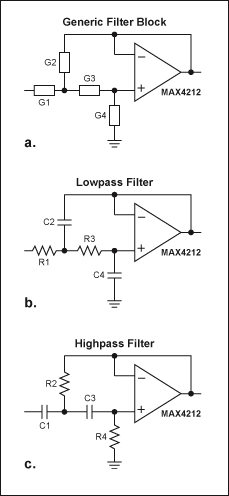The transfer function for the lowpass filter (Figure 1b) is:This equation is simpler with conductances. Replace the capacitors with a conductance of sC, and the resistors with a conductance of G. If this looks complicated, you can "normalize" the equation. Set the resistors equal to 1Ω or the capacitors equal to 1F, and change the surrounding components to fit the response. Thus, with all resistor values equal to 1Ω, the lowpass transfer function is:This transfer function describes the response of a generic, second-order lowpass filter. We now take the theoretical tables of poles that describe the three main filter responses, and translate them into real component values.

### The Design Process

To determine the type of filter required, you should use the above descriptions to select the passband performance needed. The simplest way to determine filter order is to design a 2nd-order filter stage, and then cascade multiple versions of it as required. Check to see if the result gives the desired stopband rejection, and then proceed with correct pole locations as shown in the tables in the Appendix. Once pole locations are established, the component values can soon be calculated.

First, transform each pole location into a quadratic expression similar to that in the denominator of our generic 2nd-order filter. If a quadratic equation has poles of (a ± jb), then it has roots of (s - a - jb) and (s - a + jb). When these roots are multiplied together, the resulting quadratic expression is s2 - 2as + a2 + b2.

In the pole tables, "a" is always negative, so for convenience we declare s2 + 2as + a2 + b2 and use the magnitude of "a," regardless of its sign. To put this into practice, consider a 4th-order Butterworth filter. The poles and the quadratic expression corresponding to each pole location are as follows:

 Poles (a ± jb) Quadratic Expression -0.9239 ± j0.3827 s2 + 1.8478s + 1 -0.3827 ± j0.9239 s2 + 0.7654s + 1

You can design a 4th-order Butterworth lowpass filter with this information. Simply substitute values from the above quadratic expressions into the denominator of equation 4. Thus, C2C4 = 1 and 2C4 = 1.8478 in the first filter, implying that C4 = 0.9239F and C2 = 1.08F. For the second filter, C2C4 = 1 and 2C4 = 0.7654, implying that C4 = 0.3827F and C2 = 2.61F. All resistors in both filters equal 1Ω. Cascading these two 2nd-order filters yields a 4th-order Butterworth response with rolloff frequency of 1rad/s, but the component values are impossible to find. If the frequency or component values above are not suitable, read on.

It so happens that if you maintain the ratio of the reactances to the resistors, the circuit response remains unchanged. You might therefore choose 1kΩ resistors. To ensure that the reactances increase in the same proportion as the resistances, divide the capacitor values by 1000.

We still have the perfect Butterworth response, but unfortunately the rolloff frequency is 1rad/s. To change the circuit's frequency response, we must maintain the ratio of reactances to resistances, but simply at a different frequency. For a rolloff of 1kHz rather than 1rad/s, the capacitor value must be further reduced by a factor of 2π × 1000. Thus, the capacitor's reactance does not reach the original (normalized) value until the higher frequency. The resulting 4th-order Butterworth lowpass filter with 1kHz rolloff takes the form of Figure 2.Using the above technique, you can obtain any even-order filter response by cascading 2nd-order filters. Note, however, that a 4th-order Butterworth filter is not obtained simply by calculating the components for a 2nd-order filter and then cascading two such stages. Two 2nd-order filters must be designed, each with different pole locations. If the filter has an odd order, you can simply cascade 2nd-order filters and add an RC network to gain the extra pole. For example, a 5th-order Chebyshev filter with 1dB ripple has the following poles:

 Poles Quadratic Expression -0.2265 ± j0.5918 s2 + 0.453s + 0.402 2.488s2 + 1.127s + 1 -0.08652 ± j0.9575 s2 + 0.173s + 0.924 1.08s2 + 0.187s + 1 -0.2800 see text

To ensure conformance with the generic filter described by equation 4, and to ensure that the last term equals unity, the first two quadratics have been multiplied by a constant. Thus, in the first filter C2C4 = 2.488 and 2C4 = 1.127, implying that C4 = 0.5635F and C2 = 4.41F. For the second filter, C2C4 = 1.08 and 2C4 = 0.187, implying that C4 = 0.0935F and C2 = 11.55F.

Earlier, it was shown that an RC circuit has a pole when 1 + sCR = 0: s = -1/CR. If R = 1, then to obtain the final pole at s = -0.28 you must set C = 3.57F. Using 1kΩ resistors, you can normalize for a 1kHz rolloff frequency as shown in Figure 3. Thus, designers can boldly go and design lowpass filters of any order at any frequency.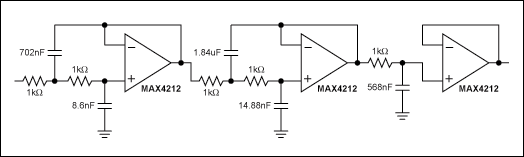All of this theory applies also to the design of highpass filters. It has been shown that a simple RC lowpass filter has the transfer function:Similarly, a simple RC highpass filter has the transfer function:Normalizing these functions to correspond with the normalized pole tables gives TF = 1/(1 + s) for lowpass and TF = 1/(1 + 1/s) for highpass.

Note that the highpass pole positions "s" can be obtained by inverting the lowpass pole positions. Inserting those values into the highpass filter block ensures the correct frequency response. To obtain the transfer function for the highpass filter block, we need to go back to the transfer function of the lowpass filter block. Thus, from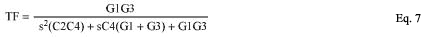we obtain the transfer function of the equivalent highpass filter block by interchanging capacitors and resistors:Again, life is much simpler if capacitors are normalized instead of resistors:Equation 9 is the transfer function of the highpass filter block. This time, we calculate resistor values instead of capacitor values. Given the general highpass filter response, we can derive the highpass pole positions by inverting the lowpass pole positions and continuing as before. Inverting a complex-pole location is easier said than done, however. As an example, consider the 5th-order, 1dB-ripple Chebyshev filter discussed earlier. It has two pole positions at (-0.2265 ± j0.5918).

The easiest way to invert a complex number is to multiply and divide by the complex conjugate, thereby obtaining a real number in the numerator. You then find the reciprocal by inverting the fraction as follows: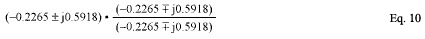Inverting this expression yields pole positions that can then be converted to the corresponding quadratic expression, and values calculated as before. The result is: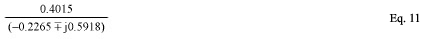Poles Quadratic Expression -0.564 ± j1.474 s2 + 1.128s + 2.490 0.401s2 + 0.453s + 1

From equation 2 we can calculate the first filter component values as R2R4 = 0.401 and 2R2 = 0.453, implying that R2 = 0.227Ω and R4 = 1.77Ω. This procedure can then be repeated for the other pole locations.

Because it has been shown that s = -1/CR, a simpler approach is to design for a lowpass filter using suitable lowpass poles and then treat every pole in the filter as a single RC circuit. To invert each lowpass pole to obtain the corresponding highpass pole, simply invert the value of CR. Once the highpass pole locations are obtained, we ensure the correct frequency response by interposing the capacitors and resistors.

A normalized capacitor value was calculated for the lowpass implementation, assuming that R = 1Ω. Hence, the value of CR equals the value of C, and the reciprocal of the value of C is the highpass pole. Treating this pole as the new value of R yields the appropriate highpass component value.

Considering again the 5th-order, 1dB-ripple Chebyshev lowpass filter, the calculated capacitor values are C4 = 0.5635F and C2 = 4.41F. To obtain the equivalent highpass resistor values, invert the values of C (to obtain highpass pole locations), and treat these poles as the new normalized resistor values: R4 = 1.77, and R2 = 0.227. This approach provides the same results as does the more formal method mentioned earlier.

Thus, the Figure 3 circuit can now be converted to a highpass filter with 1kHz rolloff by inverting the normalized capacitor values, interposing the resistors and capacitors, and scaling the values accordingly. Earlier, we divided by 2πfR to normalize the lowpass values. The scaling factor in this case is 2πfC, where C is the capacitor value and f is the frequency in hertz. The resulting circuit is Figure 4, and a SPICE simulation shows expected characteristics at the output of each filter (Figure 5).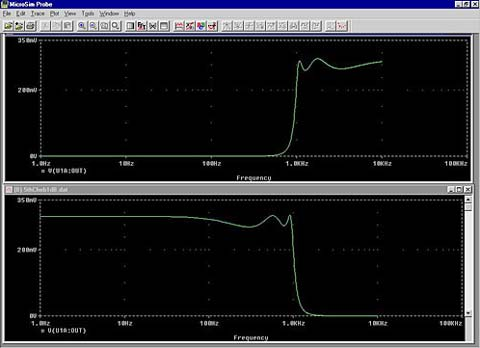### Conclusion

Using the aforementioned methods, you can design lowpass and highpass filters with response at any frequency. Bandpass and bandstop filters can also be implemented (with single op amps) using techniques similar to those shown, but those applications are beyond the scope of this article. You can, however, implement bandpass and bandstop filters by cascading lowpass and highpass filters. Information on Maxim op amps can be found at the Amplifiers and Comparators.

### Appendix

 Order Real Imaginary -a ±jb 2 0.7071 0.7071 3 0.5000 0.8660 1.0000 4 0.9239 0.3827 0.3827 0.9239 5 0.8090 0.5878 0.3090 0.9511 1.0000 6 0.9659 0.2588 0.7071 0.7071 0.2588 0.9659 7 0.9010 0.4339 0.6235 0.7818 0.2225 0.9749 1.0000 8 0.9808 0.1951 0.8315 0.5556 0.5556 0.8315 0.1951 0.9808 9 0.9397 0.3420 0.7660 0.6428 0.5000 0.8660 0.1737 0.9848 1.0000 10 0.9877 0.1564 0.8910 0.4540 0.7071 0.7071